### IMO Shortlist 1995 problem G3

Kvaliteta:
Avg: 3,5
Težina:
Avg: 7,0
The incircle of triangle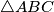$\triangle ABC$ touches the sides$BC$,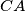$CA$,$AB$ at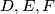$D, E, F$ respectively.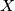$X$ is a point inside triangle of$\triangle ABC$ such that the incircle of triangle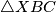$\triangle XBC$ touches$BC$ at$D$, and touches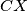$CX$ and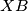$XB$ at$Y$ and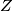$Z$ respectively.
Show that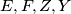$E, F, Z, Y$ are concyclic.
Izvor: Međunarodna matematička olimpijada, shortlist 1995